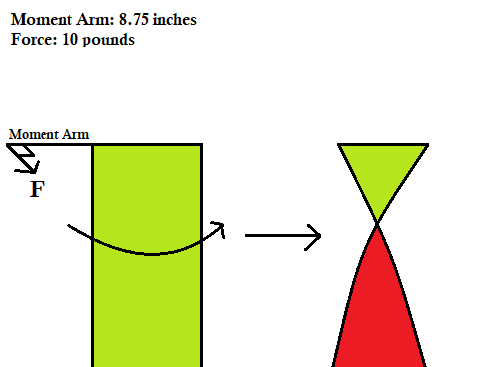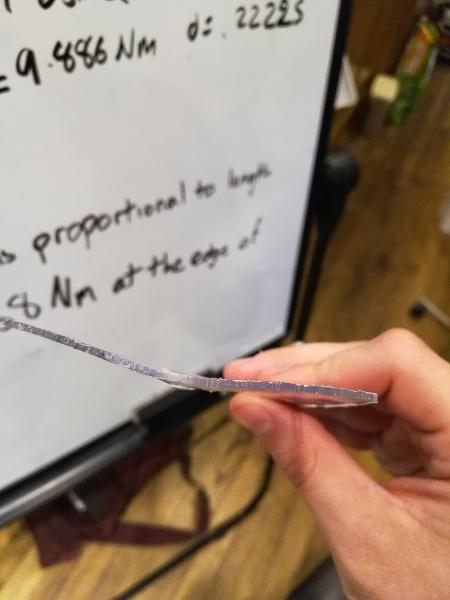# Polycarb Deformation

Tags: design and think
Personhours: 1
Polycarb Deformation By Ethan

### Task: Find a constant for polycarb deformation

Recently, we've been having an issue with our gripper in that the shielding for the sides of the intake have been bending torsionally, so that they deform and interfere with our glyph take-up. So, we created a lab to find the torque required to cause this deformation.We cut a length of polycarb with a similar width but different length to test this (thickness 3/32 of an inch), hooking it into a vertical vice. Then, we attached a vice grip of length 8.75 inches to the side, then attached various weights to the vice until the polycarb deformed.Under a ten-pound weight, the polycarb finally deformed. Using calculations, we can determine:

``` d = length of moment arm = 8.75 in = .22225 m x = 0 degrees F = 10 lbs = 44.482 N Torque = Fdsin(x) = 9.886 N*m ``` Since torque to create deformation is roughly inversely proportional to the length of any object in a single dimension (keeping thickness and width constant): ``` L' = expiremental length = 4.5 in L = actual length = 14.5 in T' = T(L'/L) = 3.068 N*m ```

This amount of torque isn't hard to generate at all, which explains why our gripper shields bend so easy. To prevent this, we must reenforce the shields with something with a higher resistance to deformation, such as thin metal strips.

### Next Steps:

We're going back and recording many of our robot's constants so that we can be better able to predict how our robot functions in various situations. This is the first of many posts.

Date | February 27, 2018